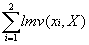We present a small example database in Table 1 to illustrate the support and confidence measures. There are five transactions in the database, so the support of each itemset is measured relative to 5.

 Transaction ID Items T1 A, C, D T2 B, E T3 A, B, C, E T4 B, E T5 B, D, F

Table 1

Table 2 shows the supports of the 6 items in the database. The first column lists the item, the second lists the number of transactions in which the item appears, and the third column lists the support of the item. Item A in the first row, for example, appears in 2 transactions in Table 1 (transactions T1 and T3). This represents 40% of the 5 total transactions.

 Item Number of transactions Support sup(X) A 2 40% B 4 80% C 2 40% D 2 40% E 3 60% F 1 20%

Table 2

Table 3 shows the support for some itemsets derived from the database. The columns are analogous to those in Table 2. For example, the itemset {A, B} in the second row appears in only one transaction, transaction T3, which gives it a support of 20%.

 Itemset Number of Transactions Support sup(X) A, C 2 40% A, B 1 20% B, D 1 20% C, D 1 20% A, B, C 1 20% A, B, E 1 20% A, C, D 1 20% B, D, F 1 20% A, B, C, E 1 20%

Table 3

Table 4 shows the confidence measures of several association rules derived from the itemsets in Table 3. The confidence of 100% for the rule A® C means that in every transaction in which A appears, C also appears. The confidence of this rule can be calculated by dividing the number of transactions in which the itemset {A, C} appears, which is 2 (see Table 3), by the number of transactions in which the item A appears, also 2 (Table 2).

 Association Rule Confidence conf(X® Y) A® C 100% A® B 50% B® D 25% A, B® C 100% A, C® B 50% B, E® A 33%

Table 4

We illustrate the share measures using the database shown in Table 5. This is the same database as shown in Table 1 except that each item has a transaction count. For this database, the set of items I = {A, B, C, D, E, F}, m = 6, and the set of transactions T = {T1, T2, T3, T4, T5}, n = 5.

 Transaction ID Item Item count T1 A 1 C 2 D 2 T2 B 1 E 3 T3 A 2 B 1 C 2 E 1 T4 B 1 E 1 T5 B 1 D 1 F 1

Table 5

Table 6 shows the transactions measure values for each item. From Table 6 we see that the set of transactions associated with the item A is TA = {T1, T3}. The global measure value for item A is:

MV(A) = tmv(A,T1) +tmv(A,T3) = 1+2 = 3.

The remaining global measure values for items B to F are listed in the rightmost column of Table 6. The total item count for the database is:

MV = MV(A) + ... + MV(F) = 20.

 Item Transaction measure values Global T1 T2 T3 T4 T5 measure values A 1 0 2 0 0 3 B 0 1 1 1 1 4 C 2 0 2 0 0 4 D 2 0 0 0 1 3 E 0 3 1 1 0 5 F 0 0 0 0 1 1

Table 6

Table 7 lists several 2-itemsets from the example database, the transactions which contain each itemset, the transaction measure values of each item and the local measure value of each itemset. If the itemset X = {A,C} (row 1 in the table), then X is associated with the set of transactions TX = {T1, T3} (see Table 5). The local count of item A in the itemset X is = tmv(A,T1) + tmv(A,T3) = 1+2 = 3 as shown in column 3. The local measure value of the item C in the itemset X is lmv(C,X) =tmv(C,T1) + tmv(C,T3) = 2+2 = 4 (column 4). The local measure value of the itemset X is therefore:

lmv(X) == 3+4 = 7

This is shown in column 5 of Table 7.

 Itemset Contained in transactions First items local measure value Second items local count Itemset local count X TX lmv(x1,X) lmv(x2,X) lmv(X) A, C {T1, T3} 3 4 7 A, B {T3} 2 1 3 B, D {T4} 1 1 2 C, D {T1} 2 2 4

Table 7